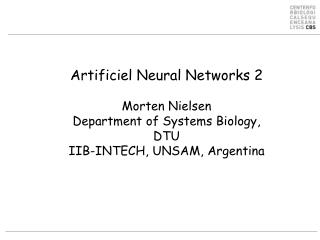DownloadDownload PresentationOutline

# Outline

Download Presentation## Outline

- - - - - - - - - - - - - - - - - - - - - - - - - - - E N D - - - - - - - - - - - - - - - - - - - - - - - - - - -
##### Presentation Transcript

1. Artificiel Neural Networks 2Morten NielsenDepartment of Systems Biology,DTUIIB-INTECH, UNSAM, Argentina

2. Outline • Optimization procedures • Gradient decent (this you already know) • Network training • back propagation • cross-validation • Over-fitting • examples

3. Neural network. Error estimate Linear function I1 I2 w1 w2 o

4. Neural networks

5. Gradient decent (from wekipedia) Gradient descent is based on the observation that if the real-valued function F(x) is defined and differentiable in a neighborhood of a point a, then F(x) decreases fastest if one goes from a in the direction of the negative gradient of F at a. It follows that, if for  > 0 a small enough number, then F(b)<F(a)

7. Gradient decent. Example Weights are changed in the opposite direction of the gradient of the error Linear function I1 I2 w1 w2 o

8. Network architecture Ik Input layer vjk hj Hidden layer Hj wj o Output layer O

9. What about the hidden layer?

10. Hidden to output layer

11. Hidden to output layer

12. Hidden to output layer

13. Input to hidden layer

14. Input to hidden layer

15. Summary

16. Or

17. Or Ii=X[k] Hj=X[j] Oi=X[i]

18. Can you do it your self? I1=1 I2=1 v21=-1 v12=1 v11=1 v22=1 h1 H1 h2 H2 w2=1 w1=-1 o O What is the output (O) from the network? What are the wij and vjk values if the target value is 0 and =0.5?

19. Can you do it your self (=0.5). Has the error decreased? After Before I1=1 I2=1 I1=1 I2=1 v21=-1 v12=1 v21= v12= v11=1 v22=1 V11= v22=. h2= H2= h1= H1= h1= H1= h2= H2= w2=1 w1=-1 w2= w1= o= O= o= O=

20. Can you do it your self? I1=1 I2=1 v21=-1 v12=1 v11=1 v22=1 h2=0 H2=0.5 h1=2 H1=0.88 w2=1 w1=-1 o=-0.38 O=0.41 • What is the output (O) from the network? • What are the wij and vjk values if the target value is 0?

21. Can you do it your self (=0.5). Has the error decreased? I1=1 I2=1 I1=1 I2=1 v21=-1 v12=1 v12=1.005 v21=-1.01 v11=1 v22=1 v22=.0.99 v11=1.005 h2=0 H2=0.5 h1=2 H1=0.88 h1=2.01 H1=0.882 h2=-0.02 H2=0.495 w2=1 w1=-1 w2=0.975 w1=-1.043 o=-0.38 O=0.41 o=-0.44 O=0.39

22. Example

23. Sequence encoding • Change in weight is linearly dependent on input value • “True” sparse encoding is therefore highly inefficient • Sparse is most often encoded as • +1/-1 or 0.9/0.05

24. Sequence encoding - rescaling • Rescaling the input values If the input (o or h) is too large or too small, g´ is zero and the weight are not changed. Optimal performance is when o and h are close to 0.5

25. Training and error reduction Size matters 

26. Example

27. Neural network training. Cross validation Cross validation Train on 4/5 of data Test on 1/5 => Produce 5 different neural networks each with a different prediction focus

28. Neural network training curve Maximum test set performance Most cable of generalizing

29. 5 fold training Which network to choose?

30. 5 fold training

31. Conventional 5 fold cross validation

32. “Nested”5 fold cross validation

33. When to be careful • When data is scarce, the performance obtained used “conventional” versus “Nested”cross validation can be very large • When data is abundant the difference is small, and “nested”cross validation might even be higher than “conventional” cross validation due to the ensemble aspect of the “nested”cross validation approach

34. NetMHCpan Do hidden neurons matter? • The environment matters

35. Context matters • FMIDWILDA YFAMYGEKVAHTHVDTLYVRYHYYTWAVLAYTWY 0.89 A0201 • FMIDWILDA YFAMYQENMAHTDANTLYIIYRDYTWVARVYRGY 0.08 A0101 • DSDGSFFLY YFAMYGEKVAHTHVDTLYVRYHYYTWAVLAYTWY 0.08 A0201 • DSDGSFFLY YFAMYQENMAHTDANTLYIIYRDYTWVARVYRGY 0.85 A0101

36. Summary • Gradient decent is used to determine the updates for the synapses in the neural network • Some relatively simple math defines the gradients • Networks without hidden layers can be solved on the back of an envelope (SMM exercise) • Hidden layers are a bit more complex, but still ok • Always train networks using a test set to stop training • Be careful when reporting predictive performance • Use “nested”cross-validation for small data sets • And hidden neurons do matter (sometimes)

37. And some more stuff for the long cold winter nights • Can it might be made differently?

38. Predicting accuracy • Can it be made differently? Reliability

39. Making sense of ANN weights • Identification of position specific receptor ligand interactions by use of artificial neural network decomposition. An investigation of interactions in the MHC:peptidesystem

40. Making sense of ANN weights

41. Making sense of ANN weights

42. Making sense of ANN weights

43. Making sense of ANN weights

44. Making sense of ANN weights

45. Making sense of ANN weights

46. Deep learning http://www.slideshare.net/hammawan/deep-neural-networks

47. Deep learning http://www.slideshare.net/hammawan/deep-neural-networks

48. Deep learning http://www.slideshare.net/hammawan/deep-neural-networks